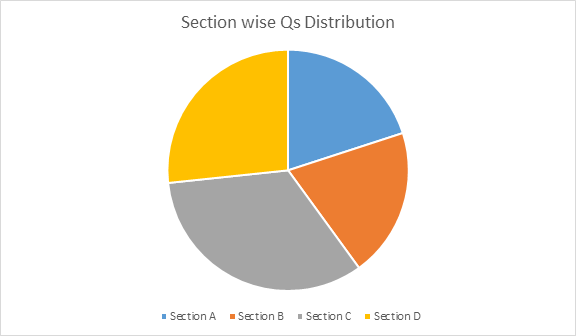CBSE Class 10 Maths Paper Analysis 2019

The CBSE Class 10 exams are going on. The class 10 science exam was conducted successfully across various exam centers by the Central Board of Secondary Education on the 13th of March, 2019. The Science question papers were distributed to the students on time, at 10:15 am, and 15 minutes were provided to read the paper. Students started writing the exam at 10:30 am. Here is the CBSE Class 10 Science Paper Analysis for the year 2019.

Visit the link below to get the detailed subject wise CBSE Paper Analysis 2019 for class 10th and 12th Board exams:

CBSE Class 10 Maths Paper 2019 Specifics:

The table below shows the specifics of CBSE Class 10 Mathematics theory paper. However, there is an internal assessment exam that conducts in school for 20 marks.

 CBSE Class 10 Maths Paper Exam Date 7th March 2019 Exam Timing 10:30 am – 1:30 pm Exam Duration 3 Hrs Maths Paper Marks Distribution 80 Marks (Theory Paper) 20 Marks (Internal Assesment)

CBSE Class 10 Maths Exam Analysis 2019

The CBSE Maths Paper consist of a total of 30 questions which were divided into 4 sections as mentioned below:

1. Section A: It carries 6 Qs with 1 marks each.
2. Section B: It carries 6 Qs with 2 marks each.
3. Section C: It carries 10 Qs with 3 marks each.
4. Section D: It carries 8 Qs with 4 marks each.

The paper was easy & most of the students could able to solve paper of 40 to 50 marks easily. Sections D was tough among all the sections whereas section C & B seems to be time-consuming for the students.CBSE Class 10 Maths Paper Mark Distribution 2019

As compared with the Previous Years, the CBSE Question paper for Class 10 Maths was comparatively easy. Some of the questions approximately 2-4 were repeated from previous years CBSE question papers.

 Section Question Type No. of Questions Total Marks Section A Very short answer Questions Consisting of 1 Marks 6 6 Section B Short Answers Consisting of 2 Marks 6 12 Section C Short Answers Consisting of 3 Marks 10 30 Section D Long Answers Consisting of 4 Marks 8 32 Total 30 80

For the Class 10 CBSE students, the next challenging paper will be science. As the science paper is on 13th March 2019, therefore, students have enough time to prepare. So utilize this time & solve Class 10 Science Previous Year Papers because some of the questions might be repeated.

Learn Maths & Science in an interactive & fun-loving way with BYJU’S App/Tablet.

All the Best for Next Paper!

Important Links to refer for upcoming CBSE Class 10 Exams:

 NCERT Related Articles NCERT Solutions for Class 12 NCERT Solutions for Class 11 NCERT Solutions for Class 10 NCERT Solutions for Class 12 Maths NCERT Solutions for Class 11 Maths NCERT Solutions for Class 10 Maths NCERT Solutions for Class 12 Physics NCERT Solutions for Class 11 Physics NCERT Solutions for Class 10 Science NCERT Solutions for Class 12 Chemistry NCERT Solutions for Class 11 Chemistry NCERT Solutions for Class 6 NCERT Solutions for Class 12 Biology NCERT Solutions for Class 11 Biology NCERT Solutions for Class 6 Maths NCERT Solutions for Class 9 NCERT Solutions for Class 8 NCERT Solutions for Class 6 Science NCERT Solutions for Class 9 Maths NCERT Solutions for Class 8 Maths NCERT Solutions for Class 4 NCERT Solutions for Class 9 Science NCERT Solutions for Class 8 Science NCERT Solutions for Class 4 Maths NCERT Solutions for Class 5 NCERT Solutions for Class 7 NCERT Solutions for Class 7 Maths NCERT Solutions for Class 4 Science NCERT Solutions for Class 5 Maths NCERT Solutions for Class 7 Science NCERT Solutions NCERT Books NCERT Solutions for Class 5 Science